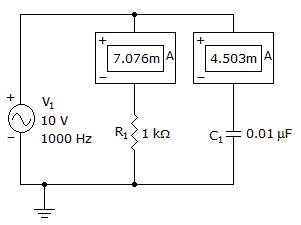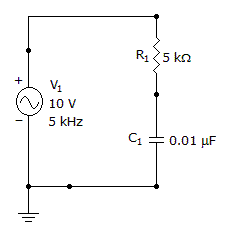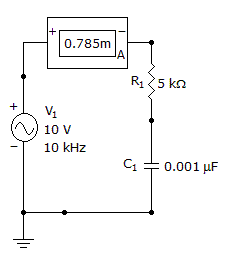# Electronics - Capacitors

### Exercise :: Capacitors - General Questions

51.

What is the circuit's impedance?A. 1192B. 843C. 723D. 511Explanation:

No answer description available for this question. Let us discuss.

52.

In an ac circuit, a capacitor and an output resistor are connected in series with an ac signal. What filter action will be the result?

 A. bandpass B. low-pass C. high-pass D. band-stop

Explanation:

No answer description available for this question. Let us discuss.

53.

What is the impedance of this circuit?A. 5928B. 2000C. 1902D. 1798Explanation:

Impedance Cannot be Directly Measured, We Need to find Xc first.

So,

Xc = 1/2πfC.

Xc = 1/( 2 x 3.14 x 5000 x 0.01 x 10-6 ).

Xc = 3184.7.

Z = Sqrt( Xc2 + R2 ).

Z = Sqrt( 3184.72 + 50002 ).

Z = 5928 Ω.

54.

What is the reactive power?A. 6.8 mW B. 9.8 mW C. 12.8 mW D. 15.8 mW

Explanation:

No answer description available for this question. Let us discuss.

55.

A practical capacitor

 A. is a short to dc B. looks like a capacitor in parallel with a resistor C. is an open to ac D. stores energy in a magnetic field